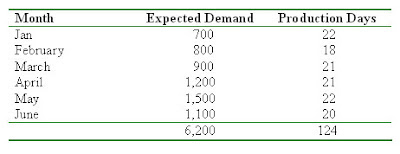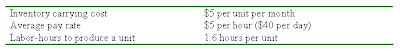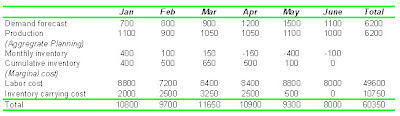## Sunday, April 15, 2007

### Management Lite & Ezy 32 – Aggregate Planning II

Part I is here.

Example

A TV manufacturer has developed monthly forecasts for the 6-month period from July to December. The forecasts are presented in the table below…Various costs involved are given in the table below… (Click for larger image.)Plan: The factory will maintain a constant workforce throughout the 6-month period, no overtime, and ending inventory = 0:

To achieve zero ending inventory, we will need to compute the average requirement.

Average requirement

= total expected demand/number of production days

= 6,200/124

= 50 units per day

We will then work out the production level, inventory, labor cost and inventory carrying cost, using the formulae given below…

Production = average requirement x number of production days

Monthly inventory = production – forecast

Cumulative inventory = inventory of this month + inventory from previous month

Labor cost

= total units x labor hours per unit x pay rate

= total units x 1.6 x 5

Inventory carrying cost

= cumulative inventory x inventory carrying cost

= cumulative inventory x 5Jay Heizer & Barry Render, “Operations Management”, 8th edition